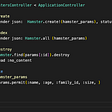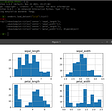# Python Programming — Conditional Statement

`x = float (input ('Enter a number = '));if(x >= 10): print ('Congratulations, the number you entered is correct')`
`Enter a number = 11Congratulations, the number you entered is correct`
`Enter a number = 9#program not executed`
`x = int(input('enter the value of x: '))y = int(input('enter the value of y: '))if x < y:                    print('x is less than y') else:                        print('x is more than y')print('x={}, y={}'.format(x, y))`
`enter the value of x: 5enter the value of y: 2x is more than yx=5, y=2`
`enter the value of x: 5enter the value of y: 5x is more than yx=5, y=5`
`x = int(input('enter the value of x: '))y = int(input('enter the value of y: '))if x < y :                    print('x is less than y') elif x > y :   print('x is more than y') else :    print('x is equal to y')    print('x={}, y={}'.format(x, y))`
`enter the value of x: 5enter the value of y: 5x is equal to yx=5, y=5`
• The conditional statement starts from the first, when a statement is fulfilled, the statement command will be executed and the conditional statement will be terminated
• Conditional expression is a conditional statement that is tested which can contain mathematical operators, functions, and logic
• Each conditional expression ends with a colon :
• Every conditional expression has a command code in writing indents
• Conditional_expression_1 is the first conditional statement with the command command_1
• The second and so on conditional expressions start with the syntax elif
• Conditional_expression_2 is the second conditional statement with the command command_2
• Conditional_expression_3 is the third conditional statement with the command command_3
• Alternative conditions are written ending with the else syntax:
• alternative_command is an alternative command, used when all conditional conditions are not met
• if you buy it will display the number of items purchased and the total price of the item
• If after buying the remaining money will be displayed in the wallet
• If you can’t buy an item, it will show that you have less money
`burger_price = 5money = 20input_count = input('How many burgers do you want : ')count = int(input_count)total_price = burger_price * countprint('You will buy ' + str(count) + ' burgers')print('Total price is ' + str(total_price) + ' dolars')if money > total_price:    print('You have bought ' + str(count) + ' burgers')    print('your money is left ' + str(money - total_price) + ' dolar')elif money == total_price:    print('You have bought ' + str(count) + ' burgers')    print('your wallet is empty')else:    print('your money is not enough')    print('you cant buy that many burgers')`
`#if can buy a burgerHow many burgers do you want : 3You will buy 3 burgersTotal price is 15 dolarsYou have bought 3 burgersyour money is left 5 dolar#if can't buy a burgerHow many burgers do you want : 10You will buy 10 burgersTotal price is 50 dolarsyour money is not enoughyou cant buy that many burgers`

--

--

--

## More from Nabil Makarim

NIM (K3521056), EMAIL : bluerejet1330@gmail.com, UNIVERSITAS SEBELAS MARET

Love podcasts or audiobooks? Learn on the go with our new app.

## Why Lazy Evaluation enhances performance and makes Apache Spark distinct?## Where To Get A Dedicated Development Team — Denmark## Multi-Region Apache Cassandra on Azure Kubernetes Service with the K8ssandra Operator (Part One)## Rails G Resource: Friend or Foe?## Revise GIT, CAP theorem and MongoDB## Factomize Core Dev Update 1 — Factomize## Nabil Makarim

NIM (K3521056), EMAIL : bluerejet1330@gmail.com, UNIVERSITAS SEBELAS MARET

## DATATYPES IN PYTHON-## Quick Python Console## Pythonic solution of the 2x2 Rubic cube## Rock Paper Scissors in Python